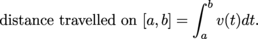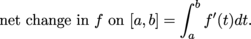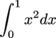Study Guide

# The Fundamental Theorem of Calculus

## The Fundamental Theorem of Calculus Introduction

The Fundamental Theorem of Calculus—or FTC if you're texting your BFF about said theorem—proves that derivatives are the yin to integral's yang. It's what makes these inverse operations join hands and skip. We thought they didn't get along, always wanting to do the opposite thing. All they needed was a little TLC from the FTC. Who knew?

The Fundamental Theorem of Calculus is what officially shows how integrals and derivatives are linked to one another. The FTC is super important—dare we say integral—when learning about definite and indefinite integrals, so give it some love. It's also one of the theorems that pops up on exams. Just saying.

To start with a realistic example, we'll check out what it means to integrate the velocity function. When velocity is constant, let's say we're driving due west at 65 MPH in search of a Sheetz MTO (or perhaps you prefer Wawa), we can find distance travelled using the formula

distance travelled = speed × time.

When the velocity v(t) is changing we can find the distance travelled using an integral instead:Generalizing to other types of functions we get the first Fundamental Theorem of Calculus, which says we can find the change in f on an interval by integrating f's rate of change:The first Fundamental Theorem of Calculus also finally lets us exactly evaluate (instead of approximate) integrals like.

There's also a second Fundamental Theorem of Calculus that tells us how to build functions with particular derivatives. We won't necessarily have nice formulas for these functions, but that's okay–we can deal.

## The Fundamental Theorem of Calculus Resources

### Websites

Fundamental Theorem of Calculus With Examples
The FTC is the rivet that binds derivatives to integrals. It's also a useful tool to cheat your way out of doing some integrals. This is one theorem you want to know.

Distance, Velocity, and Acceleration
Want to calculate how fast to throw a nerf ball at your brothers head? The laws of motion are derived here using integration.

Second Fundamental Theorem of Calculus With Examples
The FTC has a doppelganger known as the Second FTC. Check out the not-so-evil twin of the FTC and some examples on how to use it.

### Videos

The Indefinite Integral or the Antiderivative
No, the antiderivative is not an evil incarnation come to see to the destruction of mathematics. In this video, the antiderivative idea is explained as the inverse of the derivative.

Integral Involving Ice
Icebergs and calculus go together like sushi and chopsticks. You can't have one without the other.

Dr. Bob Explains the First and Second Fundamental Theorems
Pop open a Dr. Pepper and have Dr. Bob explain these two theorems to you. Take a sip every time he says, "integral".

The FTC Proved!
If a wacky waving inflatable arm flailing tube man captures your attention, this video on the proof of the FTC will do just the same.

A Word Problem with Position, Velocity, and Acceleration
Find out how to use antiderivatives to save you from running over a chupacabra. It may be more advantageous to hit it.

### Vidoes

Fundamental Theorem of Calculus Explained
With several good examples, you'll feel like a superhero with the power to solve any FTC problem after watching this video. Maybe you'll get laser beam eyes, too.

Applying the Second Fundamental Theorem of Calculus
Applying the Second FTC is a bit like swinging a fly swatter at wasp. Swing it right, and you'll get it. Swing wrong, and you'll get stung. This MIT professor shows you how to use the Second FTC.